Homework Help Question & Answers

10. A 3-phase, salient pole synchronous motor operates at rated voltage on infinite busbars. If t...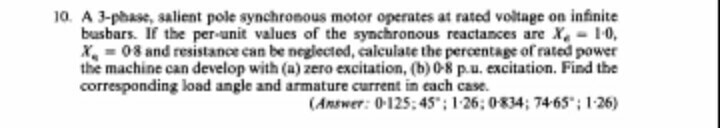10. A 3-phase, salient pole synchronous motor operates at rated voltage on infinite busbars. If the per-unit values of the synchronous reactances are X0 x08 and resistance can be neglected, calculate the percentage of rated power the machine can develop with (a) zero excitation, (b) 08 р.u. excitation. Find the corresponding load angle and armature current in each case. Answer: 0-125:45: 126; 08347465"; 1-26)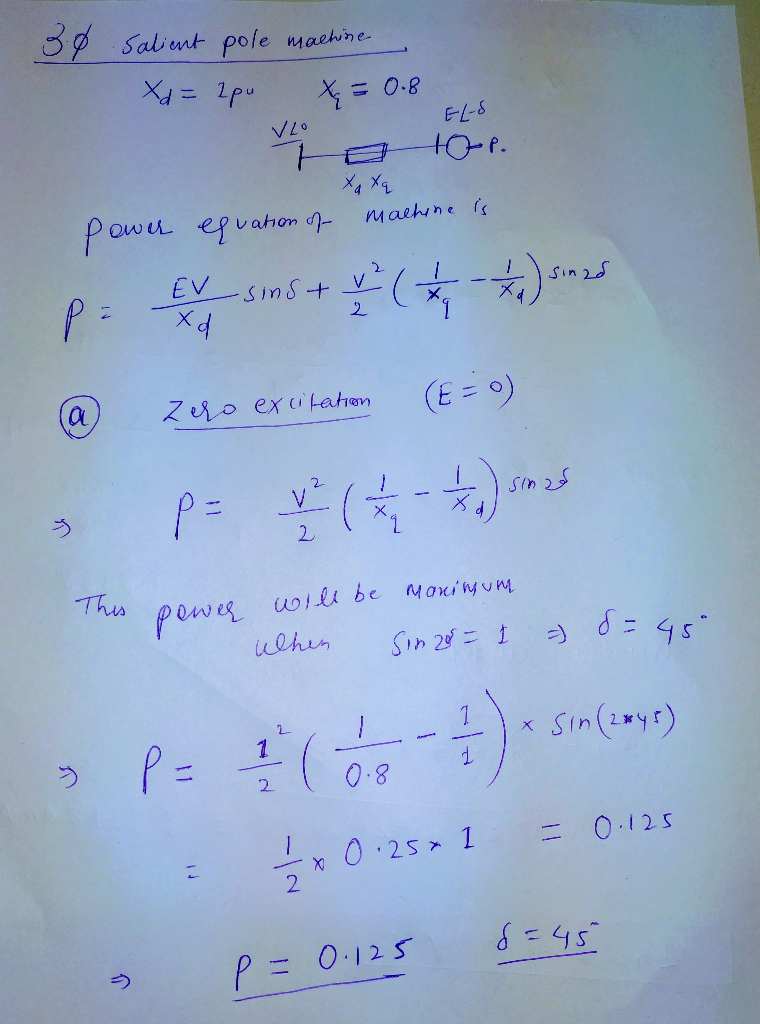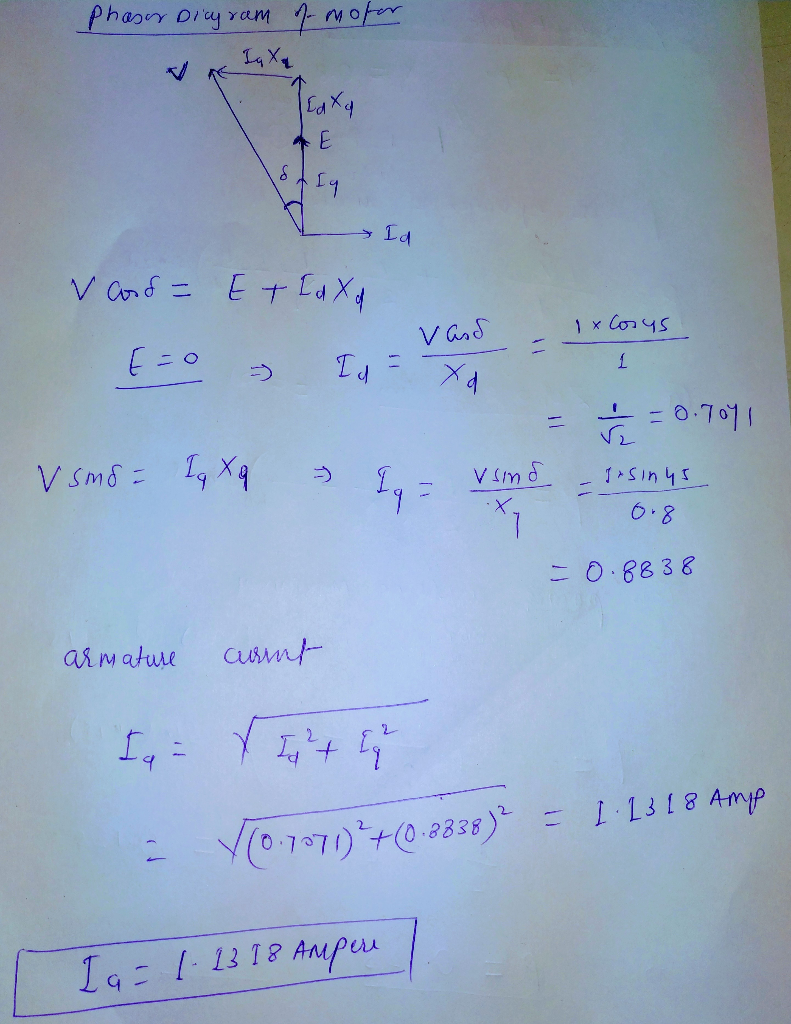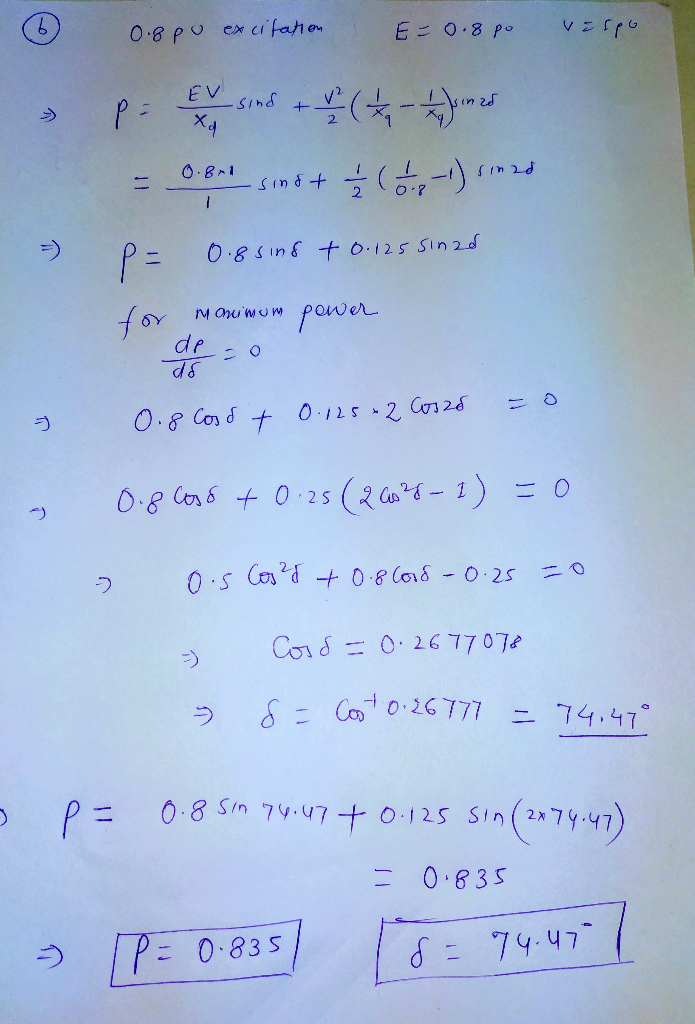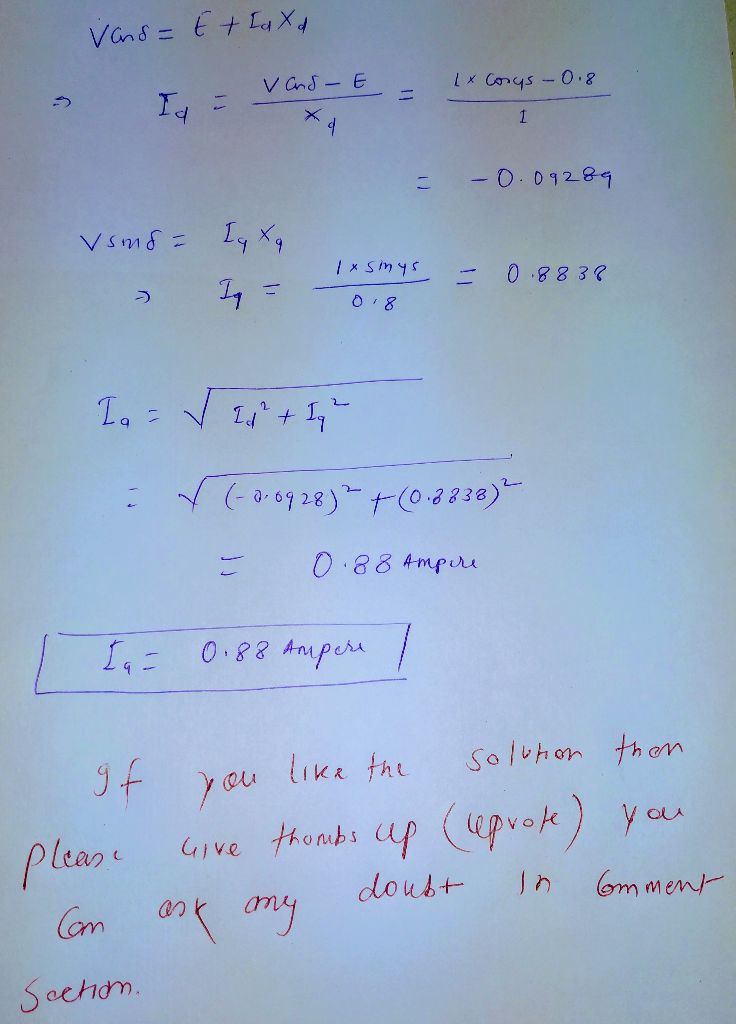Add Answer of: 10. A 3-phase, salient pole synchronous motor operates at rated voltage on infinite busbars. If t...
More Homework Help Questions Additional questions in this topic.

• 4. A 150 kVA, 460-V, four pole, 60 Hz, three phase, Y-connected synchronous motor operatingat rat...

Need Online Homework Help?

Get FREE EXPERT Answers
WITHIN MINUTES
Related Questions